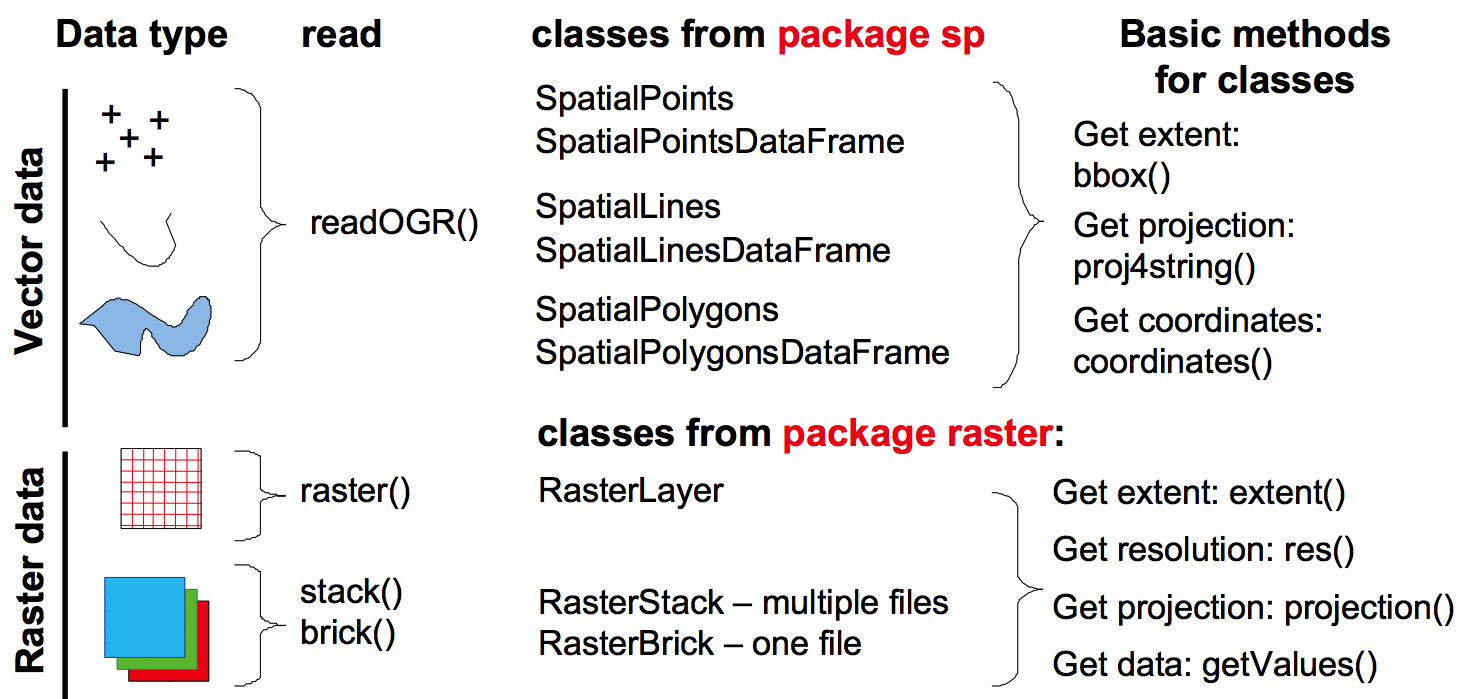## Objectives:

• Load spatial data in R (vector and raster in different formats, including NetCDF).
• Access information from spatial data (projection system, extent, attribute table)
• Create maps
• Overlay spatial data and extract information from raster and vectors

#### Get the data and R script:

2. Unzip the archive in a new folder. The zip file contains data files, R-scripts and the present document as a pdf
3. Open the R script script1_LoadExtractGIS.R with your favorite R editor (RStudio is recommended)
4. Be sure to set the working directory (Session > Set Working Directory) to the directory where the script and the data are located.

#### Load the needed packages and functions

In the following tutorial, we will use seven packages and some home-made functions saved in the file `MapTools.R`. Let’s first, load all these needed functions:

``````# To load and process GIS data
require(sp)
require(rgdal)
require(raster)
require(ncdf4)
#To make nicer looking maps
require(maps)
require(mapdata)
require(RColorBrewer)
source("MapTools.R")``````

If you have an error message, check if the packages are installed. If not, install them by typing `install.packages(c("maps", "mapdata", "ncdf4", "raster", "rgdal", "RColorBrewer", "sp"))`.
Check also that the file `MapTools.R` is in your working directory.#### Bathymetry of the Baltic Sea

The General Bathymetric Chart of the Oceans (GEBCO) provides global bathymetry dataset with a high resolution of 30 arc-second. In this example, the file GEBCO2014_Subset_30Sec.tif is a subset of the global dataset. The file is a Geotiff (an image with regular grid structure and information on projection system) and can be loaded with the function `raster`.

``````#Set the directory of the GEBCO file
dir<-"Data/GEBCO2014_Subset_30Sec.tif"
bathy <- raster(dir) ``````

``````#Projection system
proj4string(bathy)``````
`` "+proj=longlat +datum=WGS84 +no_defs +ellps=WGS84 +towgs84=0,0,0"``

Here the projection system is `longlat`, i.e. the coordinates are not projected, and expressed in degrees in latitude and longitude. The datum is `WGS84` (World Geodesic System 1984) which is the most common datum nowadays. This projection system (`+proj=longlat +datum=WGS84`) is very common for output of models and dataset with global coverage. In the following examples, all the data have the same projection, but one has to keep in mind that the coordinate reference systems is an important part of spatial data, and may be a source of error.

``````#Extent of the dataset
bbox(bathy)``````
``````   min max
s1  -5  30
s2  50  70``````

The bathymetry data covers the rectangle defined in longitude between 5\(^\circ\)W and 30\(^\circ\)E; and in latitude between 50\(^\circ\)N and 70\(^\circ\)N.

``````#Dimension and resolution
dim(bathy)``````
`` 2400 4200    1``
``res(bathy)``
`` 0.008333333 0.008333333``

The bathymetry grid has a dimension of 2400 x 4200 pixels and a resolution of 30 arc-second (= 0.00833\(^\circ\) in decimal degree).

#### Visualize

The easiest way to see a raster is to use the function `plot` from the `raster` package. Interesting features include defining the region plotted (with the arguments `xlim` and `ylim`), limiting the number of pixel to be plotted (to limit the processing time, argument `maxpixels`), choosing the color palette (argument `col`) and defining the breaks between the color classes (argument `breaks`).

Here is a simple example to show the full raster and the countries around:

``````#Visualize the raster
plot(bathy, maxpixels = 20000)